# 三种雾霾图像去雾技术分析Analysis of Image Defogging Technology for Unmanned Vehicle

DOI: 10.12677/JISP.2020.93019, PDF, HTML, XML, 下载: 24  浏览: 66

Abstract: In real life, many cities in China suffer from haze in autumn and winter. The frequent occurrence of haze weather is a serious problem for vehicle safety driving and road traffic control. Due to the scattering effect of particles in the atmosphere, the quality of the photos taken by the machine is seriously reduced, which not only affects the visual effect of the photos, but also adversely affects the extraction of key information in the images. In view of the above problems, students adopt three common defogging technologies (histogram equalization, Retinex image enhancement algorithm and dark channel prior defogging algorithm) to process the actual traffic condition pictures collected in haze days. The results were evaluated by comparing the license plate information and gray histogram of the images before and after processing.

1. 引言

2. 三种常见的图像处理方法

2.1. 全局直方图均衡化

1) 统计原始图像各灰度级的像素数目ni，0 ≤ i < L，L是图像中所有的灰度数(通常为256)；

2) 图像中灰度为i的像素的出现概率是：px(i) = p(x = i) = ni/n，n是图像中所有的像素数，px(i)实际上是像素值为i的图像的直方图，归一化到[0, 1]；

3) px的累积分布函数，是图像的累计归一化直方图：

$cd{f}_{x}\left(\text{i}\right)={\sum }_{j=1}^{i}{p}_{x}\left(i\right)$ (2.1.1)

4) 直方图均衡化计算公式，cdfmin为累积分布函数最小值，M和N分别代表了图像的长宽像素个数，而L则是灰度级数(如图像为8位深度，则灰度级别共有28 = 256级数，这也是最常见的灰度级数)，v为原始图像中为v的像素值：

$h\left(\nu \right)=round\left(\frac{cdf\left(v\right)-cd{f}_{\mathrm{min}}}{\left(M\ast N\right)-cd{f}_{\mathrm{min}}}\ast \left(L-1\right)\right)$ (2.1.2)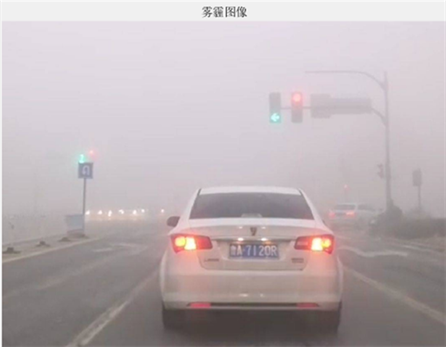Figure 1. Haze image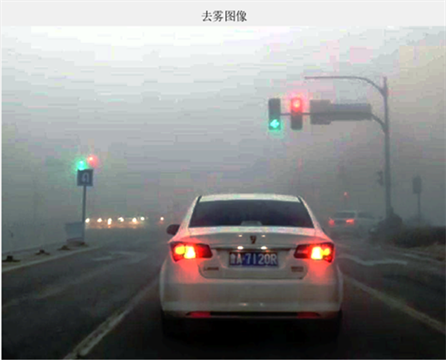Figure 2. Image after defogging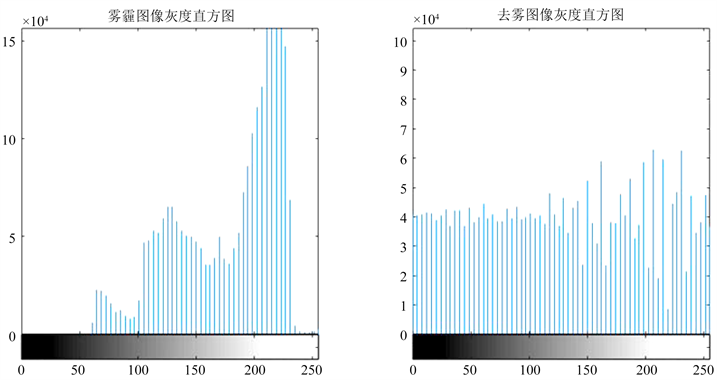Figure 3. Gray histogram of image before and after defogging

2.2. 暗原色先验去雾算法

${J}^{dark}\left(x\right)={\mathrm{min}}_{y\in \Omega \left(x\right)}\left({\mathrm{min}}_{c\in \left(r,g,b\right)}\left({J}^{c}\left(y\right)\right)\right)$ (2.2.1)

$t\left(x\right)=1-w{\mathrm{min}}_{y\in \Omega \left(x\right)}\left({\mathrm{min}}_{c\in \left(r,g,b\right)}\left(\frac{{I}^{c}\left(y\right)}{{A}^{c}}\right)\right)$ (2.2.2)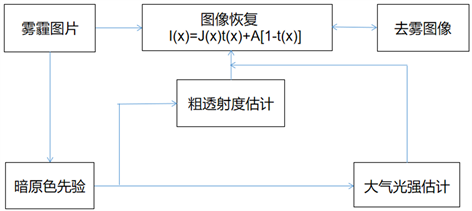Figure 4. Dark channel prior defogging flow chart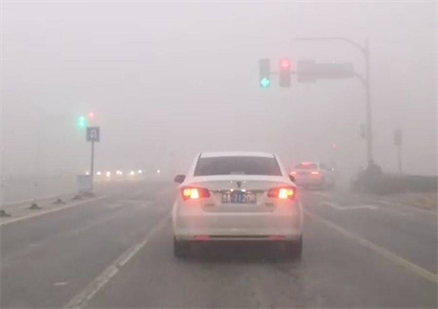Figure 5. Haze image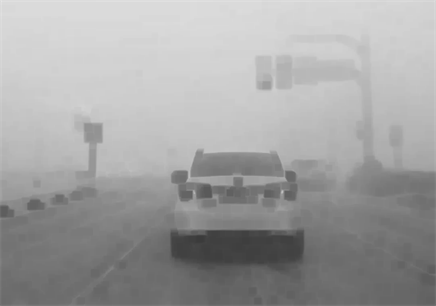Figure 6. Process 1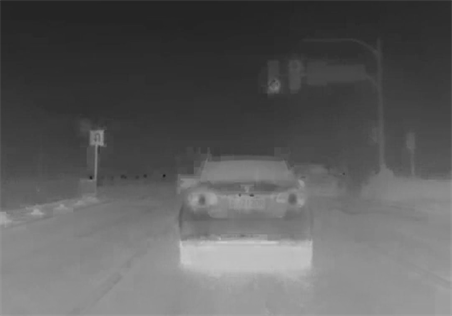Figure 7. Process 2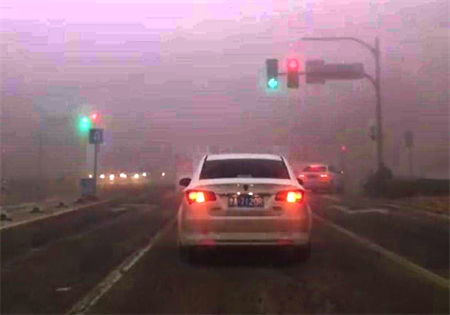Figure 8. Image after defogging

2.3. Retinex图像增强算法

$\text{I}\left(\text{x},\text{y}\right)=\text{R}\left(\text{x},\text{y}\right)\ast \text{L}\left(\text{x},\text{y}\right)$ (2.3.1)Figure 9. Retinex algorithm flow chart

$\text{L}\left(\text{x},\text{y}\right)=\text{I}\left(\text{x},\text{y}\right)\ast \text{F}\left(\text{x},\text{y}\right)$ (2.3.2)

$F\left(x,y\right)=\lambda {\text{e}}^{\frac{-\left({x}^{2}+{y}^{2}\right)}{{c}^{2}}}$ (2.3.3)

Table 1. The results under different sigma values

3. 三种去雾技术的比较

NOTES

*通讯作者。

  朱舞雪, 宋春林. 基于视频的雾天驾驶场景及其能见度识别算法研究[J]. 图像与信号处理, 2015, 4(3): 67-77. https://doi.org/10.12677/JISP.2015.43008  王宁. 雾霾对交通安全的影响分析[J]. 人民交通, 2018(13): 64-65.  何乐, 姜明新. 面向智能交通的图像去雾技术的实现[J]. 图像与信号处理, 2019, 8(3): 136-141. https://doi.org/10.12677/JISP.2019.83019  王质春, 俞文燕. 单幅雾霾天气交通监控图像去雾处理技术[J]. 交通运输研究, 2016, 2(2): 46-52.  吴成茂. 直方图均衡化的数学模型研究[J]. 电子学报, 2013, 41(3): 598-602.  单建华, 何金洪. 基于人类视觉系统的动态直方图均衡算法[J]. 计算机科学与应用, 2013, 3(2): 110-116. https://doi.org/10.12677/CSA.2013.32019  沈跃杰, 姜明新. 雾天中车牌识别系统的设计与实现[J]. 计算机科学与应用, 2019, 9(5): 985-992. https://doi.org/10.12677/CSA.2019.95111  He, K.M., Sun, J. and Tang, X.O. (2011) Single Image Haze Removal Using Dark Channel Prior. IEEE Transactions on Pattern Analysis and Machine Intelligence, 33, 2341-2353. https://doi.org/10.1109/TPAMI.2010.168  林椹尠, 张发, 李小平, 等. 基于暗原色先验的图像快速去雾[J]. 计算机应用与软件, 2020, 37(2): 171-176.  卢辉斌, 赵燕芳, 赵永杰, 温淑焕, 马金荣, Lam Hak Keung, 王洪斌. 基于亮通道和暗通道结合的图像去雾[J]. 光学学报, 2018, 38(11): 233-240.  刘健, 郭潇, 徐鑫龙, 等. 基于Retinex理论的低照度图像增强技术[J]. 火力与指挥控制, 2019, 44(9): 139-143.  贾存坤, 戴声奎, 卫志敏. 采用亮通道先验的低照度图像增强算法[J]. 华侨大学学报(自然科学版), 2018, 39(4): 595-599.